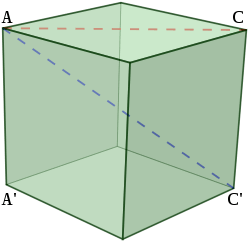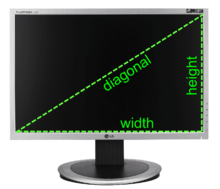# Diagonal

For the avenue in Barcelona, see Avinguda Diagonal.The diagonals of a cube with side length 1. AC' (shown in blue) is a space diagonal with length, while AC (shown in red) is a face diagonal and has length.

In geometry, a diagonal is a line segment joining two vertices of a polygon or polyhedron, when those vertices are not on the same edge. Informally, any sloping line is called diagonal. The word "diagonal" derives from the ancient Greek διαγώνιος diagonios, "from angle to angle" (from διά- dia-, "through", "across" and γωνία gonia, "angle", related to gony "knee"); it was used by both Strabo and Euclid to refer to a line connecting two vertices of a rhombus or cuboid, and later adopted into Latin as diagonus ("slanting line").

In matrix algebra, a diagonal of a square matrix is a set of entries extending from one corner to the farthest corner.

There are also other, non-mathematical uses.

## Non-mathematical usesA stand of basic scaffolding on a house construction site, with diagonal braces to maintain its structure

In engineering, a diagonal brace is a beam used to brace a rectangular structure (such as scaffolding) to withstand strong forces pushing into it; although called a diagonal, due to practical considerations diagonal braces are often not connected to the corners of the rectangle.

Diagonal pliers are wire-cutting pliers defined by the cutting edges of the jaws intersects the joint rivet at an angle or "on a diagonal", hence the name.

A diagonal lashing is a type of lashing used to bind spars or poles together applied so that the lashings cross over the poles at an angle.

In association football, the diagonal system of control is the method referees and assistant referees use to position themselves in one of the four quadrants of the pitch.The diagonal is a common measurement of display size.

## Polygons

As applied to a polygon, a diagonal is a line segment joining any two non-consecutive vertices. Therefore, a quadrilateral has two diagonals, joining opposite pairs of vertices. For any convex polygon, all the diagonals are inside the polygon, but for re-entrant polygons, some diagonals are outside of the polygon.

Any n-sided polygon (n ≥ 3), convex or concave, hasdiagonals, as each vertex has diagonals to all other vertices except itself and the two adjacent vertices, or n  3 diagonals, and each diagonal is shared by two vertices.

Sides Diagonals
3 0
4 2
5 5
6 9
7 14
8 20
9 27
10 35
Sides Diagonals
11 44
12 54
13 65
14 77
15 90
16 104
17 119
18 135
Sides Diagonals
19 152
20 170
21 189
22 209
23 230
24 252
25 275
26 299
Sides Diagonals
27 324
28 350
29 377
30 405
31 434
32 464
33 495
34 527
Sides Diagonals
35 560
36 594
37 629
38 665
39 702
40 740
41 779
42 819

### Regions formed by diagonals

In a convex polygon, if no three diagonals are concurrent at a single point, the number of regions that the diagonals divide the interior into is given byFor n-gons with n=3. 4, ... the number of regions is

1, 4, 11, 25, 50, 91, 154, 246...

This is OEIS sequence A006522.

## Matrices

In the case of a square matrix, the main or principal diagonal is the diagonal line of entries running from the top-left corner to the bottom-right corner. For a matrixwith row index specified byand column index specified by, these would be entrieswith. For example, the identity matrix can be defined as having entries of 1 on the main diagonal and zeroes elsewhere:The top-right to bottom-left diagonal is sometimes described as the minor diagonal or antidiagonal. The off-diagonal entries are those not on the main diagonal. A diagonal matrix is one whose off-diagonal entries are all zero.

A superdiagonal entry is one that is directly above and to the right of the main diagonal. Just as diagonal entries are thosewith, the superdiagonal entries are those with. For example, the non-zero entries of the following matrix all lie in the superdiagonal:Likewise, a subdiagonal entry is one that is directly below and to the left of the main diagonal, that is, an entrywith. General matrix diagonals can be specified by an indexmeasured relative to the main diagonal: the main diagonal has; the superdiagonal has; the subdiagonal has; and in general, the-diagonal consists of the entrieswith.

## Geometry

By analogy, the subset of the Cartesian product X×X of any set X with itself, consisting of all pairs (x,x), is called the diagonal, and is the graph of the equality relation on X or equivalently the graph of the identity function from X to x. This plays an important part in geometry; for example, the fixed points of a mapping F from X to itself may be obtained by intersecting the graph of F with the diagonal.

In geometric studies, the idea of intersecting the diagonal with itself is common, not directly, but by perturbing it within an equivalence class. This is related at a deep level with the Euler characteristic and the zeros of vector fields. For example, the circle S1 has Betti numbers 1, 1, 0, 0, 0, and therefore Euler characteristic 0. A geometric way of expressing this is to look at the diagonal on the two-torus S1xS1 and observe that it can move off itself by the small motion (θ, θ) to (θ, θ + ε). In general, the intersection number of the graph of a function with the diagonal may be computed using homology via the Lefschetz fixed point theorem; the self-intersection of the diagonal is the special case of the identity function.

## Notes

1. Online Etymology Dictionary
2. Strabo, Geography 2.1.36–37
3. Euclid, Elements book 11, proposition 28
4. Euclid, Elements book 11, proposition 38
5. Weisstein, Eric W. "Polygon Diagonal." From MathWorld--A Wolfram Web Resource. http://mathworld.wolfram.com/PolygonDiagonal.html
6. http://oeis.org/A006522
7. Bronson (1970, p. 2)
8. Herstein (1964, p. 239)
9. Nering (1970, p. 38)
10. Herstein (1964, p. 239)
11. Nering (1970, p. 38)
12. Bronson (1970, pp. 203,205)
13. Herstein (1964, p. 239)
14. Cullen (1966, p. 114)

## References

• Bronson, Richard (1970), Matrix Methods: An Introduction, New York: Academic Press, LCCN 70097490
• Cullen, Charles G. (1966), Matrices and Linear Transformations, Reading: Addison-Wesley, LCCN 66021267
• Herstein, I. N. (1964), Topics In Algebra, Waltham: Blaisdell Publishing Company, ISBN 978-1114541016
• Nering, Evar D. (1970), Linear Algebra and Matrix Theory (2nd ed.), New York: Wiley, LCCN 76091646Look up diagonal in Wiktionary, the free dictionary.